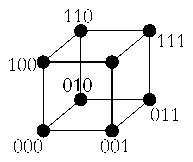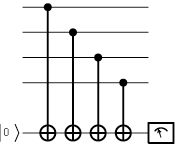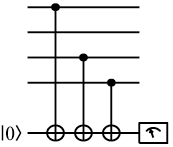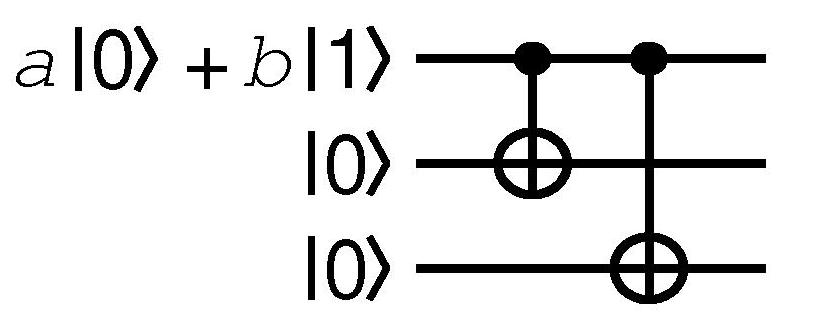4. Some ideas from classical error correction

The phrase "error correction" comes from the theory of classical information. Classical information is information that can be expressed as series of zeros and ones, i.e. bit strings. As a school boy I met the idea of error detection in the form of the "parity check bit". When a computer sends a bit string to another computer or to some other device, it often sends an additional bit called a check bit in addition to the bits (say, 1001010) to be sent. Suppose there are k bits to be sent (k=7 in our example). The sending computer sets the extra check bit to either 0 or 1, in such a way as to ensure the complete set of k+1 bits has an even number of 1's in it. In our example the complete string with check bit would be 10010101. The receiving computer (or other device) then counts the 1's in the received string. If the number of 1's is not even, it is a signal that something has gone wrong (an example of error detection). The receiving device could then for example send back a request for the message to be re-transmitted.

While playing with the idea of quantum interference involving many particles, I noticed the following. Consider a set of three two-state systems. Suppose we form the superposition state

|000> + exp(i φ ) |111> ,

and we want to observe experimentally the fact that we really have a superposition, and not just a mixture which is sometimes |000> and sometimes |111>. To do this we need some experimental signature which is sensitive to the value of φ . A convenient way to consider this is to concentrate on the two "extreme" values φ=0 and φ =π . Then the experiment must be able to distinguish (|000> + |111>) from (|000> - |111>).

In a laboratory setting, the most simple way to distinguish these states is to measure each of the qubits in the "conjugate basis" {|0">, |1">}. (In the case of spins, this means measuring the spin along a new direction; in the case of atoms it would normally be done by first rotating the atoms and then measuring them). To understand the expected measurement results, first express the state in the {|0">, |1">} basis. One finds

|000> + |111> = |000"> + |011"> +|101"> + |110">,
|000> - |111> = |001"> + |010"> +|100"> + |111">,

where for convenience we are dropping overall normalisation constants. In either case a superposition of 4 possibilities is obtained, and this means the measurement result will be one of four possible results, chosen randomly with equal probability 1/4. Each measurement result is a set of three outcomes (one for each qubit), which we write as a classical bit string. Thus in the first case, where a plus sign appears in the superposition on the left hand side (i.e. φ =0), the measurement result is one of

000 or 011 or 101 or 110.

In the second case, where a minus sign appears in the superposition on the left hand side (i.e. φ =π ), the measurement result is one of

001 or 010 or 100 or 111.

What struck me, and what I hope now strikes you, is that what distinguishes the first set of outcomes from the second set is the parity. The bit strings 000, 011, 101, 110 are the four possible 3-bit strings of even parity; the four strings 001, 010, 100, 111 are the four possible 3-bit strings of odd parity (and the combination of the first set with the second gives all possible 3-bit strings).

This observation suggests that one might like to explore a similar question for systems of larger numbers of qubits, with states such as |0000> ± |1111>, |00000> ± |11111>, |000...0> ± |111...1>. It is not hard to show that the general result holds true: the sign of the superposition in one basis is revealed by the parity of the string in the other.

After noticing this, I wondered whether I could learn some more about quantum interference of multiple particles by learning some more about classical parity checks. I had heard somewhere of something called an "error correcting code" and I remember this struck me as a wonderful-sounding phrase. It seemed to me natural that one might be able to detect errors, but strange and magical that a receiving computer could even correct them: how could it know what had gone wrong? In any case I went along to the Bodlean library in Oxford and looked for some introductory books on error correction in classical information science.I soon learned that the possibility of error correction as such is not so surprising: it can be achieved for example by a simple process of repetition (e.g. for 0 send three zeros, for 1 send three ones; if at most one bit is erroneously flipped, the receiving computer can still deduce what was sent). However, the full richness of classical error correcting codes goes well beyond this simple idea. It is a rather beautiful branch of mathematics in its own right. One of the basic concepts is to extend the notion of the parity check. As well as finding the parity of a complete bit string, one might calculate the parity of some subset of the bits, such as the first four, or some other group. For example, 00011, 01100, 00101, 111010 all have even parity overall, but 00011, 01100 also have even parity in their first three bits, while 00101, 111010 do not. Another useful idea is to imagine bit strings as if they represented vectors on a square lattice (see figure). For up to three bits, one can draw the lattice easily; for more bits one has to imagine further dimensions. The main point is that errors (bit flips) can be understood as moving around on this lattice, and the lattice gives a natural notion of "distance" between bit strings. To calculate distance we just sum the steps needed to move around the lattice; this exactly corresponds to the number of bits one would have to flip in order to move between one bit-string and another.Let's look at the idea of a parity check for the case of quantum bits. The network diagram on the left shows how to measure the overall parity of a set of qubits, without measuring anything else. The bottom qubit is introduced to facilitate things (it is called an "ancilla" from the latin for "helper"). The ancilla qubit is prepared in |0> and then a sequence of controlled-not gates from the data qubits onto the ancilla qubit are applied. These gates flip the ancilla or "check bit" between |0> and |1> either an even or an odd number of times, depending on the parity of the bit string stored in the data qubits. Then the ancilla qubit is measured. Because it is a qubit (a 2-state system) only a two-valued measurement result is possible. This two-valued result is the parity (0 signifying even, 1 signifying odd). No other information about the data qubits is revealed by this physical process, even in principle, therefore the parity is the only thing that has been measured (and thereby disturbed).This network diagram shows a parity check measurement, but now checking a subset of the data qubits rather than all of them.

Now let's introduce the simplest classical error correcting code, and apply it in a quantum setting. The simplest classical code has two "codewords", that is, legal bit strings. They are 000 and 111. A message is "encoded" by translating it into the available codewords. In this example, with only two codewords, each use of the code can only convey a two-valud message, i.e. a single classical bit. The translation or "encoding" is
0 000,
1 111.
(The terminology "encode" and "decode" has no connotation of trying to keep secrets, it just refers to the translation process and its inverse). Clearly these bit strings have different parity: they do not both satisfy an overall parity check. However there are several parity checks they do both satisfy: for any pair of bits, in either codeword, the parity is even. In the quantum case this observation is highly significant. It means that if we form a superposition

a |000> + b |111> ,

and then measure the parity of any pair of qubits, the measurement result is 0 (even parity), irrespective of the values of a and b, and furthermore no disturbance is caused to the state (because it was in fact an eigenstate of the observable being measured).The diagram on the left shows a network to take a single qubit in some general state |y> =  a |0> + b |1> , and "encode" it into a set of three qubits, producing the state

a |000> + b |111> .An uncontrolled noise process is then applied to the qubits, and after that the parity checks are measured (using two ancilla qubits, one for each parity check). If the noise caused a bit to flip, then one or both of the checks will "fail" (that is, reveal odd parity), and furthermore it is possible to deduce, from the parity check information, which bit was flipped. The parity check information thus allows one to know what corrective measure will restore the 3-qubit state to its perfect form. The measurement has revealed the error without disturbing the stored quantum information.

(A proof of the above statements may be found in a tutorial article or my early paper.)

Astute readers will realise that these parity checks cannot detect all error processes, and the interpretation of the check results is to some extent ambiguous: a flip of both the first two qubits will give the same check information as a flip of the last qubit. The latter occurs in classical error correction. One has to live with it. In practice, if the noise is not too large, it will usually flip bits one at a time rather than in pairs, so one has a good chance of interpreting the check information (called the syndrome) correctly.

The other problem, that not all error processes are even signalled by the parity checks, is more important. For qubits, it is not just flips (i.e. |0> |1> ) that matter. Rotations ("phase errors") such as

(a |0> + b |1> ) (a |0> + e b |1> ),

are just as important, and just as damaging when they are caused by some noise process. It is the correction of both bit flip errors and phase errors together which is the really crucial achievement of quantum error correction, and understanding how to do it is the goal of the rest of this introduction.